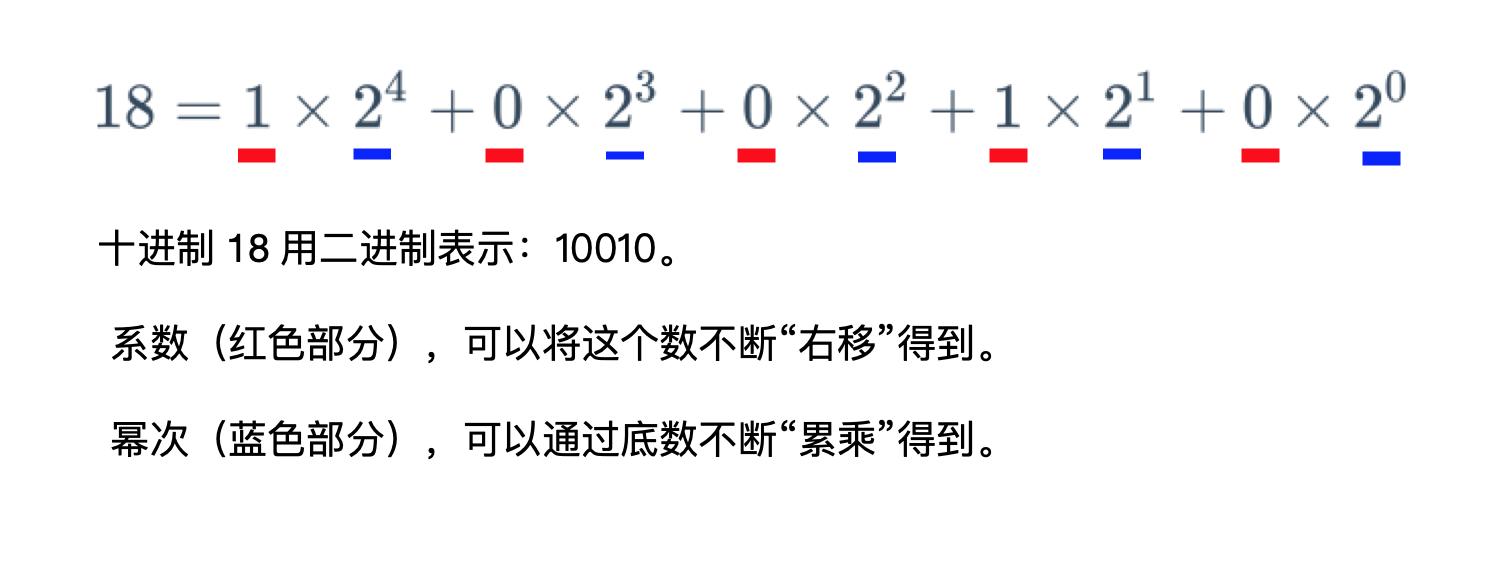# 「力扣」第 50 题：Pow(x, n)

liweiwei1419 ... 2022-1-6 位运算
• 位运算

## # 题目描述

输入：x = 2.00000, n = 10


1
2

输入：x = 2.10000, n = 3


1
2

输入：x = 2.00000, n = -2


1
2
3

• $-100.0 < x < 100.0$
• $-2^{31} \le n \le 2^{31}-1$
• $-10^4 \le x^n \le 10^4$

## # 把指数部分看做二进制数（Python 代码）

$18 = 1 \times 2^4 + 1\times2^1$

$5^{18} = 5^{2^4} \times 5^{2^1}$class Solution:
def myPow(self, x: float, n: int) -> float:

if n < 0:
x = 1 / x
n = -n

res = 1
while n:
if n & 1:
res *= x
x *= x
n >>= 1
return res

1
2
3
4
5
6
7
8
9
10
11
12
13
14
Last update: January 14, 2022 00:24
Contributors: liweiwei1419